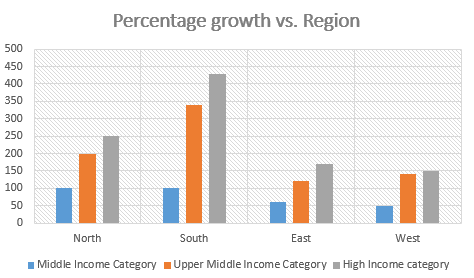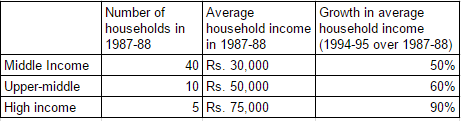### CAT 1989 Question Paper Question 10

Instructions

Answer the questions based on the following information. The following bar chart gives the growth percentage in the number of households in the middle, upper-middle and high-income categories in the four regions for the period between 1987-88 and 1994-95.(Number of households in thousands)

Question 10

# What was the average income of the high-income group in 1987-88?

Solution

The answer to this question is provided in the table.
The average income of middle income category is 30,000
The average income of upper-middle income category is 50,000 and
The average income of high income category is 75,000

Hence, the correct answer is 75,000

• All Quant CAT Formulas and shortcuts PDF
• 30+ CAT previous papers with solutions PDF